• ## 基于语音信号MFCC的情感识别

千次阅读 热门讨论 2016-06-13 22:42:05
信号处理课程结束了，没有像期望的那样学到很多东西，买的书（Discrete-Time）也没怎么看，作业算是完成了，但是要达到该坑的...基于MFCC语音情感识别在人类的面对面交流场景中，语音所传达的信息量占有很大的比重

信号处理课程结束了，没有像期望的那样学到很多东西，买的书（Discrete-Time）也没怎么看，作业算是完成了，但是要达到该坑的国际领先水平，距离有些遥远。我只是抚摸了一下信号处理的皮毛，我只是用了一下支持向量机。然而，我对语音情感的实际使用价值却没一个月之前那么看好了，或许面部表情和生理信号更加靠谱，也或许……

基于MFCC的语音情感识别
在人类的面对面交流场景中，语音所传达的信息量占有很大的比重，仅次于面部表情。基于比较成熟的信号处理技术、认知心理学模型以及计算机技术，语音情感计算模型在情感计算领域已经发展了十多年。它聚焦于语音数字信号的特征提取与分析、情感语音库的建立和语音信号的情感分类。本文的目的是给出语音情感计算的概况以及最新进展，探讨语音情感计算的整个过程。首先，介绍了语音情感计算的定义以及它的交叉学科的本质。接下来，从数字信号处理的角度研究了基于最常用的数字语音信号特征——MFCC的语音情感检测的全过程。然后，利用柏林语音情感数据库分析了在提取MFCC的过程中一些具体的参数的选择对检测结果准确率的影响。最后，阐述了语音情感计算领域面临的挑战以及今后的可能发展趋势。
用的是柏林语音情感数据库，最终的识别率勉强接近50%，存在的问题我也思考了，但是一时解决不了。

    % process
clear all;%
%cd D:\Program Files\MATLAB\R2014a\toolbox\libsvm-3.21\matlab
cd C:\Users\mayax\Desktop\现代信号处理【报告】——参考文献\samples\data\wav
file = dir('*.wav');
anger = file(1:127);
boredom = file(128:208);
disgust = file(209:254);
fear = file(255:323);
happiness = file(324:394);
neutral = file(395:473);
%读取音频原始序列并得到特征参数

for i = 1:1:length(anger)
feature_anger(i,:) = mfcc_extract_func( y,fs );
end

for i = 1:1:length(boredom)
feature_boredom(i,:) = mfcc_extract_func( y,fs );
end
for i = 1:1:length(disgust)
feature_disgust(i,:) = mfcc_extract_func( y,fs );
end
for i = 1:1:length(fear)
feature_fear(i,:) = mfcc_extract_func( y,fs );
end
for i = 1:1:length(happiness)
feature_happiness(i,:) = mfcc_extract_func( y,fs );
end
for i = 1:1:length(neutral)
feature_neutral(i,:) = mfcc_extract_func( y,fs );
end
end
%
train_matrix_size = [42,27,15,23,24,26,21];
%construct training matrix separately
training_matrix_anger = feature_anger(1:train_matrix_size(class_label('anger')),:);
training_matrix_boredom = feature_boredom(1:train_matrix_size(class_label('boredom')),:);
training_matrix_disgust = feature_disgust(1:train_matrix_size(class_label('disgust')),:);
training_matrix_fear = feature_fear(1:train_matrix_size(class_label('fear')),:);
training_matrix_happiness = feature_happiness(1:train_matrix_size(class_label('happiness')),:);
training_matrix_neutral = feature_neutral(1:train_matrix_size(class_label('neutral')),:);
%construct training label seprately
training_label_anger = class_label('anger')*ones(size(training_matrix_anger,1),1);
training_label_boredom = class_label('boredom')*ones(size(training_matrix_boredom,1),1);
training_label_disgust = class_label('disgust')*ones(size(training_matrix_disgust,1),1);
training_label_fear = class_label('fear')*ones(size(training_matrix_fear,1),1);
training_label_happiness = class_label('happiness')*ones(size(training_matrix_happiness,1),1);
training_label_neutral = class_label('neutral')*ones(size(training_matrix_neutral,1),1);
% fuse training data
%matrix
training_matrix = [training_matrix_anger; training_matrix_boredom; training_matrix_disgust; training_matrix_fear; ...
%label
training_label = [training_label_anger; training_label_boredom; training_label_disgust; training_label_fear; ...
%construct testing matrix separately
testing_matrix_anger = feature_anger(train_matrix_size(class_label('anger'))+1:end,:);
testing_matrix_boredom = feature_boredom(train_matrix_size(class_label('boredom'))+1:end,:);
testing_matrix_disgust = feature_disgust(train_matrix_size(class_label('disgust'))+1:end,:);
testing_matrix_fear = feature_fear(train_matrix_size(class_label('fear'))+1:end,:);
testing_matrix_happiness = feature_happiness(train_matrix_size(class_label('happiness'))+1:end,:);
testing_matrix_neutral = feature_neutral(train_matrix_size(class_label('neutral'))+1:end,:);
%construct testing label seprately
testing_label_anger = class_label('anger')*ones(size(testing_matrix_anger,1),1);
testing_label_boredom = class_label('boredom')*ones(size(testing_matrix_boredom,1),1);
testing_label_disgust = class_label('disgust')*ones(size(testing_matrix_disgust,1),1);
testing_label_fear = class_label('fear')*ones(size(testing_matrix_fear,1),1);
testing_label_happiness = class_label('happiness')*ones(size(testing_matrix_happiness,1),1);
testing_label_neutral = class_label('neutral')*ones(size(testing_matrix_neutral,1),1);

% fuse testing data
%matrix
testing_matrix = [testing_matrix_anger; testing_matrix_boredom; testing_matrix_disgust; testing_matrix_fear; ...
%label
testing_label = [testing_label_anger; testing_label_boredom; testing_label_disgust; testing_label_fear; ...
%training svm model
cd 'D:\Program Files\MATLAB\R2014a\toolbox\libsvm-3.21\matlab'
[testing_matrix_scale, training_matrix_scale] = scaleForSVM(testing_matrix, training_matrix,-1,1);
[testing_matrix_pca,training_matrix_pca] = pcaForSVM(testing_matrix_scale, training_matrix_scale, 90);
model = svmtrain(training_label, training_matrix_pca);
[predicted_label] = svmpredict(testing_label, testing_matrix_pca, model);
%分别统计各个情感的识别率
acc = zeros(7,7);
for i = 1:1:size(testing_label,1)
acc(testing_label(i),predicted_label(i)) = acc(testing_label(i),predicted_label(i)) + 1;
end
mfcc_extract_func：
function [ features ] = mfcc_extract_func( y,fs )
%UNTITLED3 此处显示有关此函数的摘要
%   此处显示详细说明
yy = filter([1 -0.97],1,y);
%组帧
frame_yy = enframe(yy,512,285);%对x 512点分为一帧
frame_yy_copy = frame_yy;
frame_count = size(frame_yy,1);
%加窗---给每一帧加上长度512的汉明窗。
for i=1:frame_count
win_frame_yy(i,:) = ((frame_yy(i,:))'.* hamming(512))';
end
%计算离散傅里叶变换以及信号功率
for i=1:frame_count
frame_YY(i,:) = fft((win_frame_yy(i,:))',512);
end
amp_frame_YY = abs(frame_YY);
pow_frame_YY = amp_frame_YY.^2;
%截取能量频谱的前257个
newpow_frame_YY = pow_frame_YY(:,1:257);
%mel滤波器组的设置
bank=melbankm(26,512,fs,0,0.5,'t');%Mel滤波器的阶数为26，fft变换的长度为512，采样频率为16000Hz
mel_energy = bank*newpow_frame_YY';
log_mel_energy = log10(mel_energy);
%离散余弦变换参数
for k=1:1:13
n=0:1:25;
dctcoef(k,:)=cos((2*n+1)*k*pi/(2*26));%第一阶MFCC是要被丢弃的，所以此处的变换矩阵的第一行可以随便算算，不必严格按照DCT的原理进行
end
mfcc1 = dctcoef*log_mel_energy;
mfcc2 = mfcc1(2:13,:);
mfcc = mfcc2;
mean_mfcc = mean(mfcc,2);
max_mfcc = max(mfcc,[],2);
min_mfcc = min(mfcc,[],2);
var_mfcc = var(mfcc,[],2);
%融合
features = [mean_mfcc',max_mfcc',min_mfcc',var_mfcc'];
for i = 1:1:48
if isnan(features(i)) == 1
features(i) = randi([-3,3])*abs(randi([-3,3]));
end
end
end


报告和ppt链接: http://pan.baidu.com/s/1o8Uxmwa 密码: diwc  参考资料：   MATLAB添加VOICEBOX工具箱  语音特征参数MFCC提取过程详解  mfcc详解  支持向量机的MATLAB工具箱  顶尖水平（并没有多顶尖，只是数据比较漂亮）——-Speech Emotion Recognition Using Fourier Parameters
展开全文信号处理 matlab 支持向量机
• ## 基于CNN+MFCC的语音情感识别

千次阅读 多人点赞 2019-08-26 11:53:01
... 近年来 ，随着信息技术的飞速发展 ，智能设备正在逐渐地融入到人们的日常生活当中 ，语音作为人机交互的最为便捷的方式之一 ，得到了广泛的应用。让机器听懂人类语言的同时 ，如何实现与人类有...语音情感识别...
个人博客：http://www.chenjianqu.com/
原文链接：http://www.chenjianqu.com/show-45.html

近年来 ，随着信息技术的飞速发展 ，智能设备正在逐渐地融入到人们的日常生活当中 ，语音作为人机交互的最为便捷的方式之一 ，得到了广泛的应用。让机器听懂人类语言的同时 ，如何实现与人类有感情的自然交流 ，是无数科研工作者的目标。语音情感识别的主要内容就是建立一种能够从语音中分析和识别人类情感的计算系统 ，实现人与机器的人性化交流。
语音情感识别的主要任务是将蕴含在语音中的情感信息提取出来并识别出其类别。目前对于情感的描述主要有两种方法。第一种是基于离散的情感划分，将人类日常生活中广泛使用的基本情感分为愤怒、开心、兴奋、悲伤、厌恶等；另一种是基于连续维度情感划分，主要通过不同的效价度和激活程度来对不同情感进行区分的。
那么作为一个分类任务，特征选择是最关键的一步。本文中使用的语音特征是梅尔倒谱系数，有关梅尔倒谱系数是什么和怎样提取的知识，可参阅文章《Python语音信号处理》。
本文在一定程度上参考了MITESHPUTHRANNEU/Speech-Emotion-Analyzer这个项目，下面开始介绍如何通过卷积神经网络进行语音情感分析。

神经网络结构
使用到的架构其实还是很简单的，如下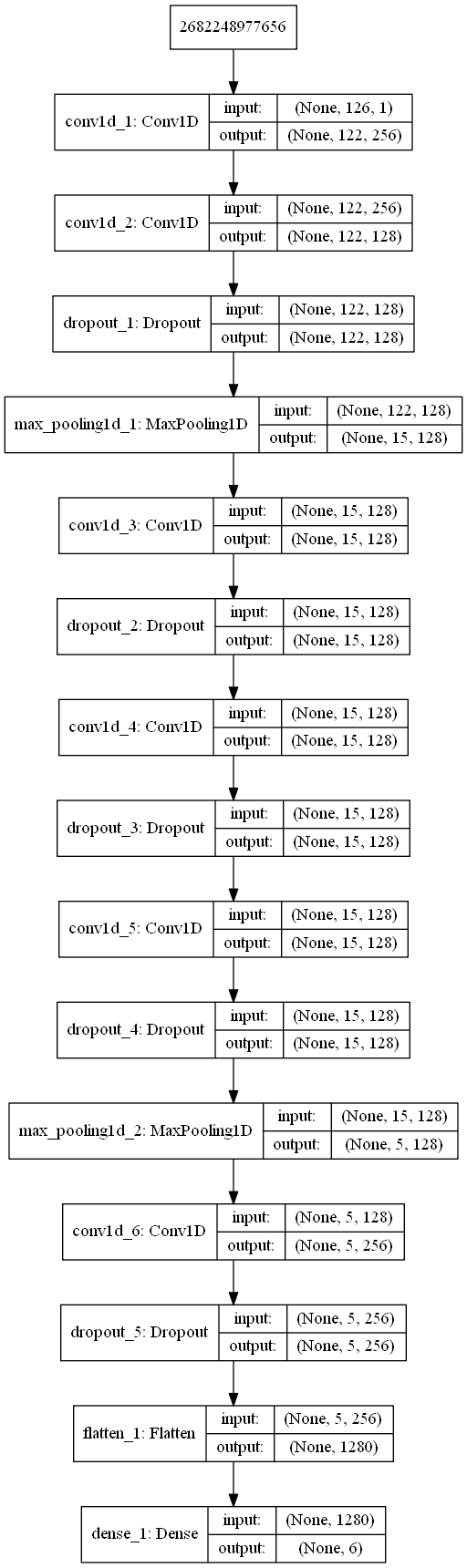数据集
但是完整的CASIA数据集是收费的，因此我只找到了1200句残缺数据集。我把我找到的数据集放在我的网盘上：https://pan.baidu.com/s/1EsRoKaF17Q_3s2t7OMNibQ。

特征提取
我使用librosa模块进行MFCC的提取，提取代码如下。
%matplotlib inline
import librosa
import matplotlib.pyplot as plt
import numpy as np

path=r'D:\NLP\dataset\语音情感\test.wav'

def normalizeVoiceLen(y,normalizedLen):
nframes=len(y)
y = np.reshape(y,[nframes,1]).T
#归一化音频长度为2s,32000数据点
if(nframes<normalizedLen):
res=normalizedLen-nframes
res_data=np.zeros([1,res],dtype=np.float32)
y = np.reshape(y,[nframes,1]).T
y=np.c_[y,res_data]
else:
y=y[:,0:normalizedLen]
return y

def getNearestLen(framelength,sr):
framesize = framelength*sr
#找到与当前framesize最接近的2的正整数次方
nfftdict = {}
lists = [32,64,128,256,512,1024]
for i in lists:
nfftdict[i] = abs(framesize - i)
sortlist = sorted(nfftdict.items(), key=lambda x: x)#按与当前framesize差值升序排列
framesize = int(sortlist)#取最接近当前framesize的那个2的正整数次方值为新的framesize
return framesize

VOICE_LEN=32000
#获得N_FFT的长度
N_FFT=getNearestLen(0.25,sr)
#统一声音范围为前两秒
y=normalizeVoiceLen(y,VOICE_LEN)
print(y.shape)
#提取mfcc特征
mfcc_data=librosa.feature.mfcc(y=y, sr=sr,n_mfcc=13,n_fft=N_FFT,hop_length=int(N_FFT/4))

# 画出特征图，将MFCC可视化。转置矩阵，使得时域是水平的
plt.matshow(mfcc_data)
plt.title('MFCC')
上面代码的作用是加载声音，取声音的前两秒进行情感分析。getNearestLen()函数根据声音的采样率确定一个合适的语音帧长用于傅立叶变换。然后通过librosa.feature.mfcc()函数提取mfcc特征，并将其可视化。
下面的代码将数据集中的mfcc特征提取出来，并对每帧的mfcc取平均，将结果保存为文件。
#提取特征
import os
import pickle

counter=0
fileDirCASIA = r'D:\NLP\dataset\语音情感\CASIA database'

mfccs={}
mfccs['angry']=[]
mfccs['fear']=[]
mfccs['happy']=[]
mfccs['neutral']=[]
mfccs['surprise']=[]
mfccs['disgust']=[]

listdir=os.listdir(fileDirCASIA)
for persondir in listdir:
if(not r'.' in persondir):
emotionDirName=os.path.join(fileDirCASIA,persondir)
emotiondir=os.listdir(emotionDirName)
for ed in emotiondir:
if(not r'.' in ed):
filesDirName=os.path.join(emotionDirName,ed)
files=os.listdir(filesDirName)
for fileName in files:
if(fileName[-3:]=='wav'):
counter+=1
fn=os.path.join(filesDirName,fileName)
print(str(counter)+fn)
y=normalizeVoiceLen(y,VOICE_LEN)#归一化长度
mfcc_data=librosa.feature.mfcc(y=y, sr=sr,n_mfcc=13,n_fft=N_FFT,hop_length=int(N_FFT/4))
feature=np.mean(mfcc_data,axis=0)
mfccs[ed].append(feature.tolist())

with open('mfcc_feature_dict.pkl', 'wb') as f:
pickle.dump(mfccs, f)
数据预处理
代码如下：
%matplotlib inline
import pickle
import os
import librosa
import matplotlib.pyplot as plt
import numpy as np
from keras import layers
from keras import models
from keras import optimizers
from keras.utils import to_categorical

#读取特征
mfccs={}
with open('mfcc_feature_dict.pkl', 'rb') as f:

#设置标签
emotionDict={}
emotionDict['angry']=0
emotionDict['fear']=1
emotionDict['happy']=2
emotionDict['neutral']=3
emotionDict['surprise']=5

data=[]
labels=[]
data=data+mfccs['angry']
print(len(mfccs['angry']))
for i in range(len(mfccs['angry'])):
labels.append(0)

data=data+mfccs['fear']
print(len(mfccs['fear']))
for i in range(len(mfccs['fear'])):
labels.append(1)

print(len(mfccs['happy']))
data=data+mfccs['happy']
for i in range(len(mfccs['happy'])):
labels.append(2)

print(len(mfccs['neutral']))
data=data+mfccs['neutral']
for i in range(len(mfccs['neutral'])):
labels.append(3)

labels.append(4)

print(len(mfccs['surprise']))
data=data+mfccs['surprise']
for i in range(len(mfccs['surprise'])):
labels.append(5)

print(len(data))
print(len(labels))

#设置数据维度
data=np.array(data)
data=data.reshape((data.shape,data.shape,1))

labels=np.array(labels)
labels=to_categorical(labels)

#数据标准化
DATA_MEAN=np.mean(data,axis=0)
DATA_STD=np.std(data,axis=0)

data-=DATA_MEAN
data/=DATA_STD
接下来保存好参数，模型预测的时候需要用到。
paraDict={}
with open('mfcc_model_para_dict.pkl', 'wb') as f:
pickle.dump(paraDict, f)
最后是打乱数据集并划分训练数据和测试数据。
ratioTrain=0.8
numTrain=int(data.shape*ratioTrain)
permutation = np.random.permutation(data.shape)
data = data[permutation,:]
labels = labels[permutation,:]

x_train=data[:numTrain]
x_val=data[numTrain:]
y_train=labels[:numTrain]
y_val=labels[numTrain:]

print(x_train.shape)
print(y_train.shape)
print(x_val.shape)
print(y_val.shape)

定义模型
使用keras定义模型，代码如下：
from keras.utils import plot_model
from keras import regularizers

model = models.Sequential()

plot_model(model,to_file='mfcc_model.png',show_shapes=True)
model.summary()
训练模型
编译并训练模型
opt = optimizers.rmsprop(lr=0.0001, decay=1e-6)
model.compile(loss='categorical_crossentropy', optimizer=opt,metrics=['accuracy'])
import keras
callbacks_list=[
keras.callbacks.EarlyStopping(
monitor='acc',
patience=50,
),
keras.callbacks.ModelCheckpoint(
filepath='speechmfcc_model_checkpoint.h5',
monitor='val_loss',
save_best_only=True
),
keras.callbacks.TensorBoard(
log_dir='speechmfcc_train_log'
)
]
history=model.fit(x_train, y_train,
batch_size=16,
epochs=200,
validation_data=(x_val, y_val),
callbacks=callbacks_list)
model.save('speech_mfcc_model.h5')
model.save_weights('speech_mfcc_model_weight.h5')
可视化训练结果：
plt.plot(history.history['loss'])
plt.plot(history.history['val_loss'])
plt.title('model loss')
plt.ylabel('loss')
plt.xlabel('epoch')
plt.legend(['train', 'test'], loc='upper left')
plt.show()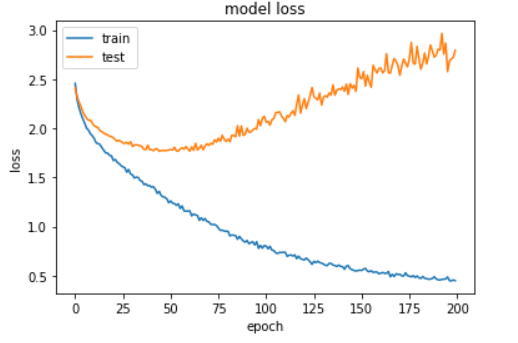plt.plot(history.history['acc'])
plt.plot(history.history['val_acc'])
plt.title('model acc')
plt.ylabel('acc')
plt.xlabel('epoch')
plt.legend(['train', 'test'], loc='upper left')
plt.show()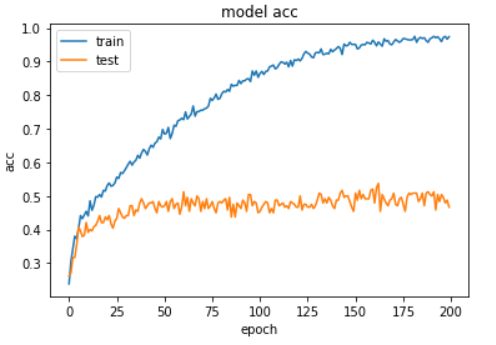从上图中可以发现，模型在训练了60轮后开始过拟合，此时训练精度达到70%，验证精度达到50%。最终训练到200轮后，训练精度达到95%。

测试
最后对训练好的模型进行测试。
#单元测试,载入模型
from keras.models import load_model
import pickle

with open('mfcc_model_para_dict.pkl', 'rb') as f:
edr = dict([(i, t) for t, i in emotionDict.items()])
import librosa

filePath=r'record1.wav'
y=normalizeVoiceLen(y,VOICE_LEN)#归一化长度
mfcc_data=librosa.feature.mfcc(y=y, sr=sr,n_mfcc=13,n_fft=N_FFT,hop_length=int(N_FFT/4))
feature=np.mean(mfcc_data,axis=0)
feature=feature.reshape((126,1))
feature-=DATA_MEAN
feature/=DATA_STD
feature=feature.reshape((1,126,1))
result=model.predict(feature)
index=np.argmax(result, axis=1)
print(edr[index])
由于数据集太小的原因，效果也就那样。

参考文献
马树文.深度学习在语音情感识别中的应用与分析.信息科技探索
展开全文Keras 卷积神经网络 情感识别
• Mel频率倒谱系数(MFCC)则是利用它们间的这种关系，计算得到的Hz频谱特征，MFCC已经广泛地应用在语音识别领域。由于Mel频率与Hz频率之间非线性的对应关系，使得MFCC随着频率的提高，其计算精度随之下降。因此，在应用...
• 本源程序代码的目的是给出语音情感计算的概况以及最新进展，探讨语音情感计算的整个过程。首先，介绍了语音情感计算...用的是柏林语音情感数据库，最终的识别率勉强接近50%，存在的问题我也思考了，但是一时解决不了。
背景
NLP和图像比较普遍，语音识别文字做的很多，今天尝试下做语音情感识别。
语音情感识别系统图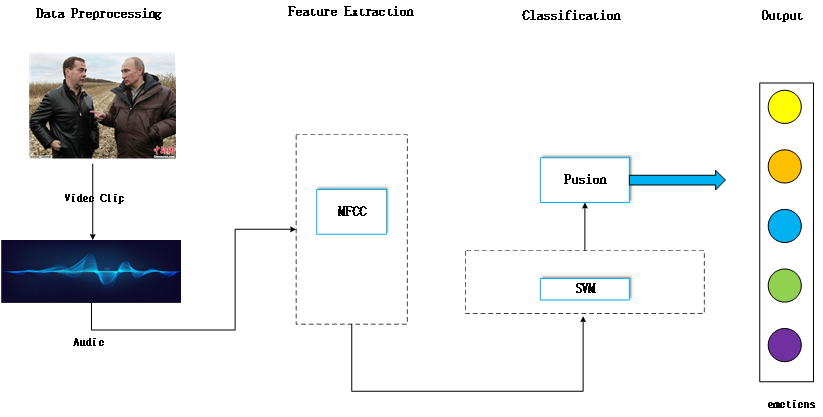wav格式的语音包
做特征提取的语音包（包含中文和英文）
特征提取方法-mfcc
在这里我选用了mfcc算法来提取语音基于谱的特征 算法如下 mfcc算法
主函数调用
#!/usr/bin/env python
# -*- coding: utf-8 -*-
'''
@File  : main.py
@Author: Piepis
@Date  : 2021/3/11 12:53
@Desc  :
'''
from calcmfcc import *
import scipy.io.wavfile as wav
import numpy
import os
import scipy.io as io

file_path = '../标准语音测试包/中文/'
mat_path="mfcc_mat_save_path.mat"
pathnames = []
for (dirpath, dirnames, filenames) in os.walk(file_path):
for filename in filenames:
if os.path.splitext(filename)[-1] ==".wav":
pathnames += [os.path.join(dirpath, filename)]

mat= numpy.zeros((len(pathnames),39*5))  #生成一个3行4列全部元素为0的矩阵
N = 0
for file in pathnames:
mfcc_feat = calcMFCC_delta_delta(sig,rate)
# axis=0，计算每一列的均值
c1=numpy.mean(mfcc_feat,axis=0) #平均值
c2=numpy.min(mfcc_feat,axis=0)  # 最小值
c3=numpy.max(mfcc_feat,axis=0)  # 最大值
c4=numpy.median(mfcc_feat,axis=0)  # 中值
c5=numpy.std(mfcc_feat,axis=0)    # 标准偏差
tt=numpy.concatenate((c1,c2 ,c3,c4,c5),axis=0)
mat[N,:]=tt
N+=1
io.savemat(mat_path, {'mfcc_name': mat})
print(mfcc_feat.shape)
print(mat.shape)


分类算法 svm
因为用来train的语音样本数据比较少，大概有一千条左右（数据来自CCPR），选用了常用于小样本的SVM算法来做情感分类。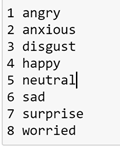核心代码
def svm_cross_validation(train_x, train_y):
from sklearn.model_selection import GridSearchCV
from sklearn.svm import SVC
model = SVC(kernel='rbf', probability=True,random_state=seed)
param_grid = {'C': [1e-3, 1e-2, 1e-1,2,0.4, 1, 10, 100, 1000], 'gamma': [0.001, 0.0001]}
grid_search = GridSearchCV(model, param_grid, n_jobs = 8, verbose=1)
grid_search.fit(train_x, train_y)
best_parameters = grid_search.best_estimator_.get_params()
for para, val in list(best_parameters.items()):
print(para, val)
model = SVC(kernel='rbf', C=best_parameters['C'], gamma=best_parameters['gamma'], probability=True)
model.fit(train_x, train_y)
return model

预测结果 与实际结果对比
没有咋找最参数，只是简单的找了下。效果不太好，40%的识别率。(也有可能是语音是从电视剧里截出来的，有很多背景杂音。我用德国语音库测了下，能达到65%以上)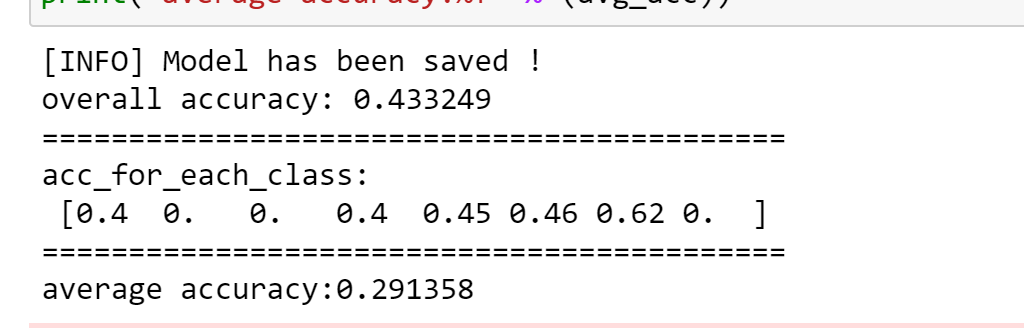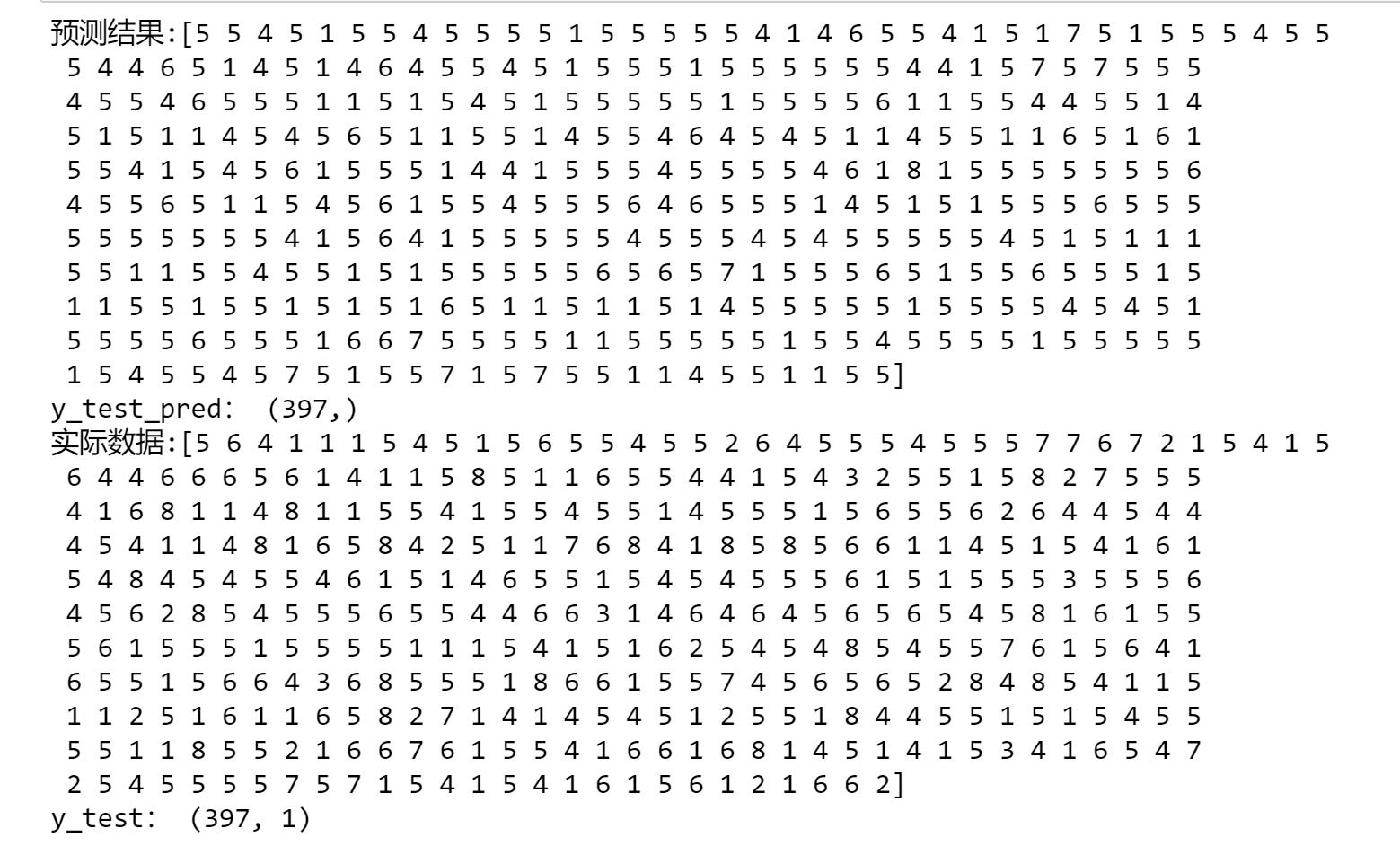混淆矩阵（Confusion Matrix）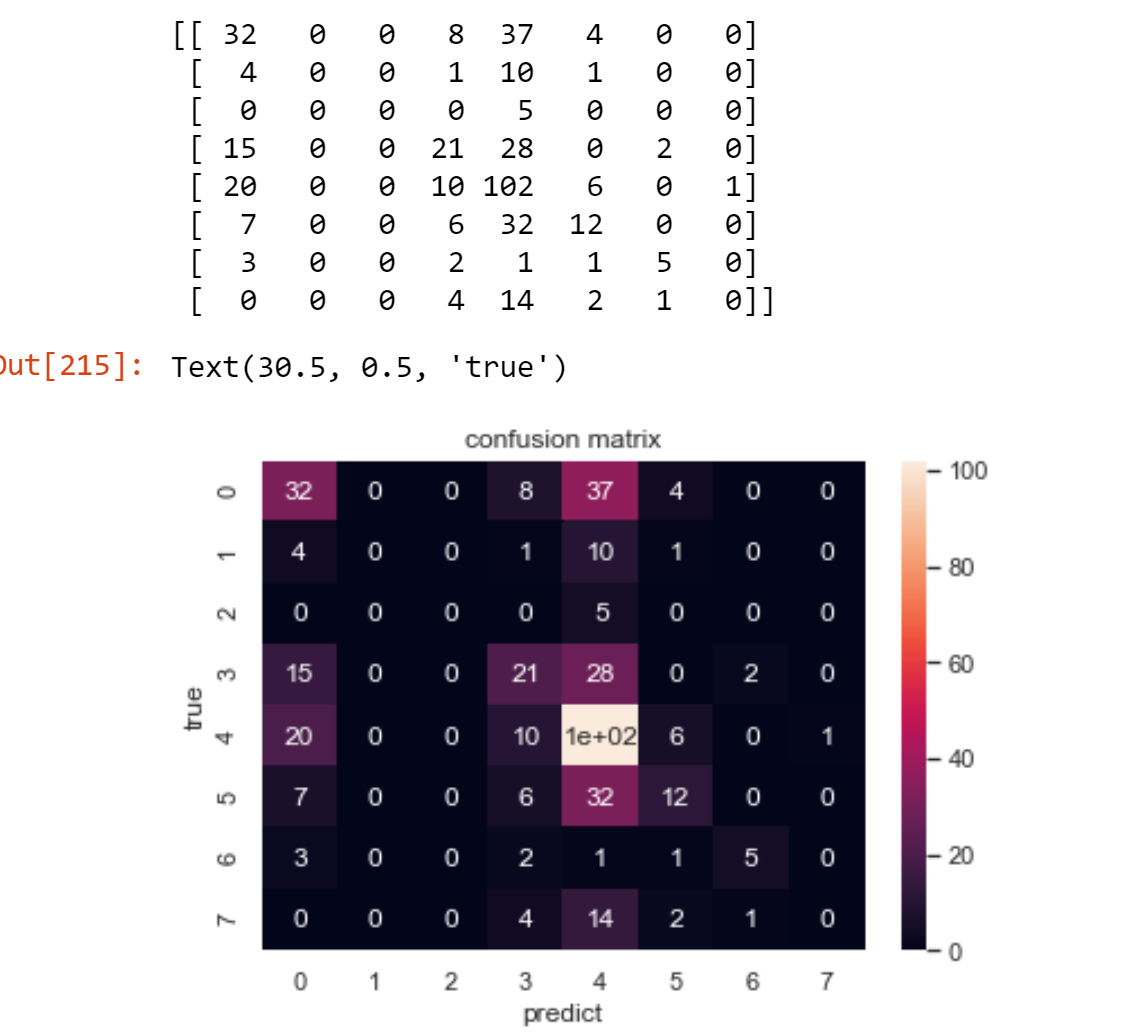参考文献
基于听觉特性的Mel频率倒谱分析 MFCC算法的实现过程（原理篇） 语音识别的第一步MFCC特征提取代码（Python）
展开全文机器学习 特征提取 svm
• ## 语音情感识别综述

千次阅读 2020-10-12 19:46:33
文献阅读语音情感识别研究进展综述（2013）摘要介绍语音情感描述模型情感语音数据库语音情感特征提取语音情感识别算法总结 语音情感识别研究进展综述（2013） 论文：语音情感识别研究进展综述 作者：韩文静 1, ...


文献阅读
语音情感识别研究进展综述（2013）摘要介绍语音情感描述模型情感语音数据库语音情感特征提取语音情感识别算法总结

语音情感识别研究进展综述（2013）
论文：语音情感识别研究进展综述 作者：韩文静 1, 李海峰 1, 阮华斌 2, 马 琳 1
摘要
对语音情感识别的研究现状和进展进行了归纳和总结,对未来语音情感识别技术发展趋势进行了展望. 从 5 个角度逐步展开进行归纳总结,即情感描述模型,具有代表性的情感语音库,语音情感特征提取,语音情感识 别算法研究和语音情感识别技术应用
介绍
自动语音情感识别则是计算机对人类情感感知和理解过程的模拟,它的任务就是从采集到的语音信号中提取表达情感的声学特征,并找出这些声学特征与人类情感的映射关系示；计算机的语音情感识别能力是计算机情感智能的重要组成部分,是实现自然人机交互界面的关键前提,具有很大的研究价值和应用价值。一般说来,语音情感识别系统主要由 3 部分组成:语音信号采集、情感特征提取和情感识别,系统框图如图1 所示.语音信号采集模块通过语音传感器(例如,麦克风等语音录制设备)获得语音信号,并传递到下一个情感特征提取模块对语音信号中与话者情感关联紧密的声学参数进行提取,最后送入情感识别模块完成情感的判断.需要特别指出的是,一个完整的语音情感识别系统除了要完善上述 3 部分以外,还离不开两项前期工作的支持:(1) 情感空间的描述;(2) 情感语料库的建立.情感空间的描述有多重标准,例如离散情感标签、激励-评价-控制空间和情感轮等,不同的标准决定了不同的情感识别方式,会对情感语料的收集标注、识别算法的选择都产生影响.情感语料库更是语音情感识别研究的基础,负责向识别系统提供训练和测试用语料数据.国内外相关研究根据研究者的出发点不同会各有侧重,但归根结底都可以涵盖到上述 5 个关键模块之中。下面从情感描述模型、情感语音 数据库、语音情感相关声学特征提取、语音情感识别算法、语音情感识别技术应用这 5 个角度对当前的语音情感识别技术主流方法和前沿进展进行系统的总结和分析。语音情感描述模型
离散形式情感描述模型 将情感描述为离散的、形容词标签的形式,如高兴、愤怒等。丰富的语言标签描述了大量的情感状态，而用于研究的情感状态需要更具普遍性，因此人们定义了基本情感类别便于研究。其中,美国心理学家 Ekman 提出的 6 大基本情感(又称为 big six)在当今情感相关研究领域的使用较为广泛。维度形式情感描述模型（连续情感描述模型） 将情感状态描述为多维情感空间中的点.这里的情感空间实际上是一个笛卡尔空间,空间的每一维 对应着情感的一个心理学属性(例如,表示情感激烈程度的激活度属性以及表明情感正负面程度的效价属性).理论上,该空间的情感描述能力能够涵盖所有的情感状态.换句话说,任意的、现实中存在的情感状态都可以在情感空间中找到相应的映射点,并且各维坐标值的数值大小反映了情感状态在相应维度上所表现出来的强弱程度。.一些既简单又能被广泛使用的维度情感描述模型有二维的激 活度-效价空间理论(arousal-valence space)、三维的激励-评 估 - 控制空间理论 (valence-activation-dominance space)和情感轮理论(emotion wheel)等情感语音数据库
用于语音情感识别的声学特征大致可归纳为韵律学特征、基于谱的相关特征和音质特征这 3 种类型
语音情感特征提取
韵律学特征： 韵律是指语音中凌驾于语义符号之上的音高、音长、快慢和轻重等方面的变化,是对语音流表达方式的一种结构性安排。它的情感区分能力已得到语音情感识别领域研究者们的广泛认可,使用非常普遍[28−31],其中最为常用的韵律特征有时长(duration)、基频(pitch)、能量(energy)等.学者们还针对韵律特征与特定情感类型之间的关联上展开了研究[3,19,35−38],这些研究工作进一步验证了韵律特征区分情感的性能,但也出现了一些不甚一致甚至相反的结论，所以，韵律特征区的情感区分能力是十分有限的.例如,愤怒、害怕、高兴和惊奇的基频特征具有相似的表现[3,36].  基于谱的相关特征 基于谱的相关特征被认为是声道(vocal tract)形状变化和发声运动(articulator movement)之间相关性的体现。Nwe 等人通过对情感语音的相关谱特征进行研究发现,语音中的情感内容对频谱能量在各个频谱区间的分布有着明显的影响.例如,表达高兴情感的语音在高频段表现出高能量,而表达悲伤的语音在同样的频段却表现出差别明显的低能量。 在语音情感识别任务中使用的线性谱特征(linear-based spectral feature) 一般有 :LPC(linear predictor coefficient),OSALPC(one-sided autocorrelation linear predictor coefficient),LFPC(log-frequency power coefficient)等;倒谱特征(cepstral-based spectral feature)一般有: LPCC(linear predictor cepstral coefficient),OSALPCC(cepstral-based OSALPC),MFCC(mel-frequency cepstral coefficient)等.  声音质量特征 声音质量是人们赋予语音的一种主观评价指标,用于衡量语音是否纯净、清晰、容易辨识等.对声音质量产生影响的声学表现有喘息、颤音、哽咽等,并且常常出现在说话者情绪激动、难以抑制的情形之下。在语音情感识别研究中,用于衡量声音质量的声学特征一般有:共振峰频率及其带宽(format frequency and bandwidth)、频率微扰和振幅微扰(jitter and shimmer)、声门参数(glottal parameter)等。 研究：.Li 等人提取了频率微扰和振幅微扰作为声音质量参数对SUSAS 数据库中的语料数据进行了说话人不相关的情感识别,HMM(hidden Markov model)被作为识别器.与仅使用 MFCC 的基线性能 65.5%相比,MFCC 和频率微扰的特征组合可以得到 68.1%的识别率,MFCC 和振幅微扰 的特征组合可以得到 68.5%的识别率,最佳性能 69.1%由 MFCC、频率微扰和振幅微扰的共同组合获得.  融合特征 上述 3 种特征分别从不同侧面对语音情感信息进行表达,自然会想到使用它们的融合用于语音情感的识别,从而达到提高系统识别性能的目的。 例如：Schuller 等人将过零率、能量、基频、声音质量、谐波噪声比、0~15 阶 MFCC 等特征的5 967 维相关统计量用于 eNTERFACE、柏林情感语料库 EMO-DB以及合成语料库的交叉数据库情感识别研究;Malandrakis 等人使用基频、强度、对数能量、过零率、频谱重心(spectral centroid)、频谱通量(spectral flux)、MFCC、PLPC(perceptual linear prediction coefficient)等特征的统计值用于电影维度情感的跟踪等。  基于 i-vector 的特征 i-vecotr 在近些年来的说话人识别领域有着广泛的应用,是一项将高维高斯混合模型(Gaussian mixture models,简称 GMM)超向量空间映射到低维总变异空间的技术。 注：特征提取的工具 openSMILE。openSMILE是一款以命令行形式运行的工具，通过配置config文件，主要用于提取音频特征， 2.0版本之后的openSMILE包括了openCV库，可以用于视频处理和视频特征提取。其中包含默认特征集及其配置文件，通用配置文件，色度特征，MFCC特征，PLP特征，韵律特征，情感识别特征提取。
语音情感识别算法
当今语音情感识别系统所采用的识别算法可以分为如下两类：离散语音情感分类器，维度语音情感分类器。
离散语音情感分类器 它们一般被建模为标准的模式分类问题,即使用标准的模式分类器进行情感的识别.常用于语音情感识别领域的分类器,线性:朴素贝叶斯Naïve Bayes Classifier,线性人工神经网络Linear ANN(artificial neural network),线性支持向量机Linear SVM(support vector machine)等;非线性: 决策树Decision Trees,k-NN(k-nearest neighbor algorithm),非线性人工神经网络Non-linear ANN,非线性支持向量机Non-linear SVM,高斯混合模型GMM (Gaussian mixture model),隐马尔可夫模型HMM (hidden Markov model)以及稀疏表示分类器等 1.2. 常用模型 HMM：隐马尔科夫模型（Hidden Markov Model，HMM），和回归、分类那些处理相互独立的样本数据的模型不同，它用于处理时间序列数据，即样本之间有时间序列关系的数据。隐藏变量是HMM里的关键概念之一，可以理解为无法直接观测到的变量，即HMM中Hidden一词的含义；与之相对的是观测变量，即可以直接观测到的变量；HMM的能力在于能够根据给出的观测变量序列，估计对应的隐藏变量序列是什么，并对未来的观测变量做预测。 GMM：GMM 是一种用于密度估计的概率模型,可以被看作是只包含一个状态的连续 HMM 模型。 ANN：MLP(multi-layer perceptron)是语音情感识别中应用最为广泛的一种人工神经网络模型,这与 MLP 完善的工具包支撑和成熟的学习算法有着很大的关系。 SVM：SVM 分类器的关键在于核函数的运用,它负责将原始特征以非线性的方式映射到高维空间中,从而提高数据的可分性。 稀疏表示分类器：稀疏表示分类器则是近年来随着压缩感知技术的兴起发展而来的一项分类技术。维度语音情感分类器 该研究一般被建模为标准的回归预测问题,即使用回归预测算法对情感属性值进行估计,在当前的维度语音情感识别领域使用较多的预测算法有:Linear Regression,k-NN,ANN,SVR(support vector regression)等.其中,SVR 因为性能稳定、训练时间短等优点应用得最为广泛。
总结
本文在充分调研和深入分析的基础上对当今的语音情感识别领域研究进展进行了综述,其中重点介绍了语音情感识别研究中的几个关键问题,包括情感描述模型选取、情感语音数据库建立、语音情感相关声学特征提取、语音情感识别算法建模等。 情感语料问题：即由于情感本身的复杂性,使得情感语音数据的采集和整理工作非常困难,进而导致了高质量的情感语料难以获取，对离散情感语音数据库而言,如何同时满足语料的自然度和情感的纯净度是其面临的最大挑战。对维度情感语音数据库的建立而言,困难不在于语料的获取,而在于语料的整理和情感的标注.为了将语料中的情感量化为精确的实数值,标注者担负了繁重的听辨和打分工作,并且标注结果的好坏、正误也难以评判。研究延伸：如何对现有资源进行补充和丰富?能否通过技术手段对训练语料的选择进行系统的指引和帮助?都是研究者们亟待解决的实际问题. 情感与声学特征之间的关联问题：计算机与人脑的情感识别机制的最初差异就是情感相关声学特征的提取以及情感与声学特征之间的关联方式的确定.因此,如果计算机不能准确地或者以尽可能接近人类的方式对情感语音进行声学特征提取并加以正确的关联和映射,就会使得计算机语音情感识别系统被建立于一个偏离实际的基础之上,从而导致其后的识别机制与人脑处理机制间的差距越来越大,无法达到期望的效果。目前性能较好的特征提取方法是Mel 倒谱系数。研究延伸：如何界定情感声学特征的最优提取时长,抑或是对不同时长的声学特征进行融合,也都是不容忽略的研究课题. 语音情感识别的建模问题：构建合理、高效的语音情感识别模型是语音情感识别研究的重中之重,它负责对大量的训练语料进行学习,从中挖掘由各种声学特征通往对应情感状态的映射通路,从而实现对测试语料情感状态的正确判断与识别。研究延伸，如何在现有的认知科学水平之上,以尽可能贴近人脑情感处理机制的方式来构建语音情感识别模型,是一项艰巨却有着重大意义的任务.
展开全文• 对中科大发布的中文语音情感数据库CASIA，提取了MFCC特征，过零率等特征，采用SVM分类，识别率很低，只适合初学者了解语音情感识别过程
• 语音情感识别（四）语音特征之声谱图，log梅尔谱，MFCC，deltas https://www.cnblogs.com/liaohuiqiang/p/10159429.html 2.1. CMU课程Speech Technology: A Practical Introduction Topic: Spectrogram,python
• 针对语音情感的动态特性, 利用动态递归Elman神经网络实现语音情感识别系统。通过连接记忆上时刻状态与当前网络一并输入, 实现Elman网络模型的状态反馈。基于此设计了语音情感识别系统, 该系统能在后台修改网络类型, ...
• 语音情感识别主要包括语音语料库的采集，语音信号预处理，语音情感特征提取，语音情感分类。以上为语音情感识别主要步骤。 语音情感特征提取(1)： 选择和提取合适的语音情感特征对提高识别准确率来说非常重要。目前...理论篇
• 通过对语音数据进行特征提取，运用SVM识别算法，实现语音信号的6种情感识别。语料库选自CASIA汉语情感语料库，特征集选取基因频率、时长、共振峰、MFCC等。
• 语音情感识别，最好的例子可以在呼叫中心看到。如果您注意到了，呼叫中心的员工永远不会以相同的方式交谈，他们与客户的推销/交谈方式会随客户而改变。现在，普通民众也确实会发生这种情况，但这与呼叫中心有何关系...python 人工智能
• 语音中准确的情绪识别对于智能医疗、智能...本文探讨了如何提高语音情感识别的准确性，包括语音信号特征提取和情感分类方法。从语音样本中提取五种特征：梅尔频率倒谱系数（mfcc）、音调、共振峰、短期过零率和短期能量
• 近来，已经对用于语音情感识别的和声特征进行了研究。 在我们的研究中发现，和声特征的一阶和二阶差异在语音情感识别中也起着重要作用。 因此，我们提出了一种新的傅立叶参数模型，该模型使用语音质量的感知内容以及...
• 不管是情感语音识别，还是语音情感识别，含义是一样的，都是对带有情感的语音信号进行情感的正确判断。最传统的情感语音识别是基于机器学习下的有监督学习完成的。有监督，即：使用训练集训练出一个模型，然后使用该...
• 语音识别MFCC特征提取matlab代码。 「梅尔倒频谱系数」（Mel-scale Frequency Cepstral Coefficients，简称MFCC），是最常用到的语音特征，此参数考虑到人耳对不同频率的感受程度，因此特别适合用在语音辨识。
• 从音频文件中读取出来的原始语音信号通常称为raw waveform，是一个一维数组，长度是由音频长度和采样率决定，比如采样率Fs为16KHz，表示一秒钟内采样16000个点，这个时候如果音频长度是10秒，那么raw waveform中就有...
• 自动语音情感识别则是计算机对人类上述情感感知和理解过程的模拟,它的任务就是从采集到的语音信号中提取表达情感的声学特征,并找出这些声学特征与人类情感的映射关系.计算机的语音情感识别能力是计算机情感智能的...
• 提取语音信号的mfcc参数用于支持向量机学习，最后识别特定语句（例如“你哈后”、“对不起”、“再见”）
• 本发明涉及语音情感识别技术领域，尤其涉及情感感知谱特征的语音情感识别方法。 背景技术 语音是人们交流中最重要的方式，语音信号不仅蕴含了丰富的语义信息，还携带了丰富的情感状态。分析...
• 支持向量机(Support Vector Machine)是Cortes和Vapnik于1995年首先提出的，它在解决小样本、非线性及高维模式识别中表现出许多特有的优势，并能够推广应用到函数拟合等其他机器学习问题中。 1 数学部分 1.1 二维空间...matlab 人工智能
• 一、BP神经网络语音情感识别简介 0 引言 随着科技的迅速发展, 人机交互显得尤为重要。语音是语言的载体, 是人与人之间交流的重要媒介。相较于其它交流方式而言, 语音交流更加直接、便捷。近年来, 随着人机交互研究的...神经网络 matlab
• 语音信号（声音是什么） 声音是由物体振动产生的声波，是通过介质（空气或固体、液体）传播并能被人或动物听觉器官所感知的波动现象，最初发出振动的物体叫声源。声音（语音消息）的基本模拟形式是一种称为语音信号...
• 支持向量机(Support Vector Machine)是Cortes和Vapnik于1995年首先提出的，它在解决小样本、非线性及高维模式识别中表现出许多特有的优势，并能够推广应用到函数拟合等其他机器学习问题中。 1 数学部分 1.1 二维空间...matlab 人工智能
• 支持向量机(Support Vector Machine)是Cortes和Vapnik于1995年首先提出的，它在解决小样本、非线性及高维模式识别中表现出许多特有的优势，并能够推广应用到函数拟合等其他机器学习问题中。 1 数学部分 1.1 二维空间...matlab 人工智能...